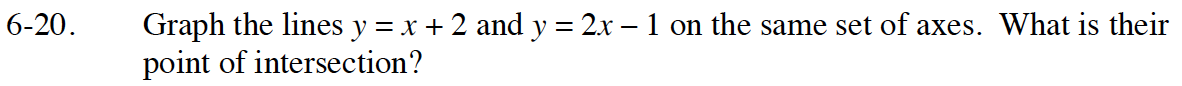Home > GB8I > Chapter cc46 > Lesson cc46.1.2 > Problem6-20

6-20.

Graph the lines y = x + 2 and y = 2x − 1 on the same set of axes. What is their point of intersection? Desmos Graphing Calculator Homework Help ✎Use the eTool below to answer this by entering one linear equation in line 1 and the other in line 2 of the Desmos calculator.
Click the link to the right to view the full version. Desmos Graphing Calculator